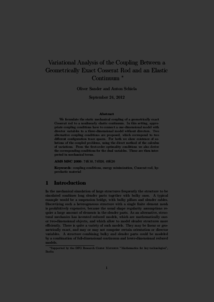Repository: Freie Universität Berlin, Math Department

# Variational analysis of the coupling between a geometrically exact Cosserat rod and an elastic continuum

Sander, O. and Schiela, A. (2014) Variational analysis of the coupling between a geometrically exact Cosserat rod and an elastic continuum. Zeitschrift für Angewandte Mathematik und Physik (ZAMP), 65 (6). pp. 1261-1288. ISSN 0044-2275 (Print) 1420-9039 (Online)Preview

475kB

Official URL: https://dx.doi.org/10.1007/s00033-013-0389-y

## Abstract

We formulate the static mechanical coupling of a geometrically exact Cosserat rod to a nonlinearly elastic continuum. In this setting, appropriate coupling conditions have to connect a one-dimensional model with director variables to a three-dimensional model without directors. Two alternative coupling conditions are proposed, which correspond to two different configuration trace spaces. For both we show existence of solutions of the coupled problems, using the direct method of the calculus of variations. From the first-order optimality conditions we also derive the corresponding conditions for the dual variables. These are then interpreted in mechanical terms.

Item Type: Article Mathematical and Computer Sciences > Mathematics > Numerical Analysis Department of Mathematics and Computer Science > Institute of Mathematics 1862 Ekaterina Engel 30 Mar 2016 17:50 03 Mar 2017 14:42

Repository Staff Only: item control page